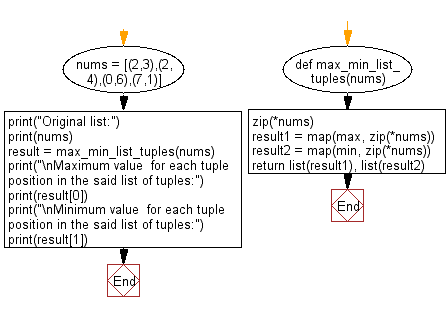﻿ Python: Find the minimum, maximum value for each tuple position in a given list of tuples - w3resource# Python: Find the minimum, maximum value for each tuple position in a given list of tuples

## Python List: Exercise - 175 with Solution

Write a Python program to find the minimum, maximum value for each tuple position in a given list of tuples.

Sample Solution:

Python Code:

``````def max_min_list_tuples(nums):
zip(*nums)
result1 = map(max, zip(*nums))
result2 = map(min, zip(*nums))
return list(result1), list(result2)

nums = [(2,3),(2,4),(0,6),(7,1)]
print("Original list:")
print(nums)

result = max_min_list_tuples(nums)
print("\nMaximum value  for each tuple position in the said list of tuples:")
print(result)
print("\nMinimum value  for each tuple position in the said list of tuples:")
print(result)
```
```

Sample Output:

```Original list:
[(2, 3), (2, 4), (0, 6), (7, 1)]

Maximum value  for each tuple position in the said list of tuples:
[7, 6]

Minimum value  for each tuple position in the said list of tuples:
[0, 1]
```

Flowchart:## Visualize Python code execution:

The following tool visualize what the computer is doing step-by-step as it executes the said program:

Python Code Editor:

Have another way to solve this solution? Contribute your code (and comments) through Disqus.

What is the difficulty level of this exercise?

Test your Python skills with w3resource's quiz

﻿

## Python: Tips of the Day

Floor Division:

When we speak of division we normally mean (/) float division operator, this will give a precise result in float format with decimals.

For a rounded integer result there is (//) floor division operator in Python. Floor division will only give integer results that are round numbers.

```print(1000 // 300)
print(1000 / 300)```

Output:

```3
3.3333333333333335```